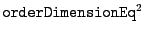Next: Interval Newton Up: Rouche theorem Previous: Mathematical background   Contents

Implementation

Rouche theorem is implemented in the following way:

• Rouche theorem is checked with respect to the mid-point of a box
• if Roucche theorem is satisfied, then a limited number of Newton iteration is performed to check if Newton indeed converge. If this is the case a ball that include a single solution has been determined
• if a ball has been determined, then, optionaly an inflation procedure )see section 3.1.6) is used to try to enlarge the ball
The syntax of the procedure is:

int Rouche(int DimensionEq,int DimVar,int order,
INTERVAL_VECTOR (* TheIntervalFunction)(int,int,INTERVAL_VECTOR &),
INTERVAL_VECTOR (* Jacobian)(int, int, INTERVAL_VECTOR &),
INTERVAL_MATRIX (* Gradient)(int, int, INTERVAL_VECTOR &),
INTERVAL_VECTOR (* OtherDerivatives)(int, int, INTERVAL_VECTOR &),
double Accuracy,
int MaxIter,
INTERVAL_VECTOR &Input,
INTERVAL_VECTOR &UnicityBox)
where
• DimensionEq: number of equations
• DimVar: number of variables
• order: the order for Rouche theorem minus 1
• TheIntervalFunction: a procedure in MakeF format for computing an interval evaluation of the equations
• Jacobian: a procedure in MakeF format that computes the jacobian row by row
• Gradient: a procedure that compute the jacobian in MakeJ format
• OtherDerivatives: a procedure in MakeF format that computes the derivative of order larger or equal to 2, row by row. This procedure returns an interval vector of dimensionThe parameters Accuracy is used in the Newton scheme to determine if Newton has converged i.e. if the residues are lower than Accuracy. A maximum of MaxIter iterations are performed. The solution found with Newton is stored in ALIAS_Simp_Sol_Newton_Numerique while a copy of the unicity box is available in ALIAS_Simp_Sol_Newton

If a ball with a single solution has been found it will be returned in UnicityBox and the procedure returns 1, otherwise it returns 0.

If the flag ALIAS_Always_Use_Inflation is set to 1, then an inflation procedure is used to try to enlarge the box up to the accuracy ALIAS_Eps_Inflation.Next: Interval Newton Up: Rouche theorem Previous: Mathematical background   Contents
Jean-Pierre Merlet 2012-12-20